Skip to main content

# 4.4: β-Hydride Insertion and Beta-Hydride Elimination

Like a coordinated carbon monoxide molecule, a coordinated organic carbonyl is also electrophilic. This time, the oxygen can be thought of as having a formal positive charge because it is donating a lone pair to a metal.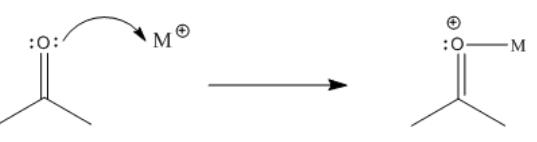Figure $$\PageIndex{1}$$: Activation of an organic carbonyl by a metal ion.

Consequently, the carbonyl is activated. It becomes a better electrophile and is more likely to undergo an addition reaction, if there are any nucleophiles nearby that are able to donate a pair of electrons.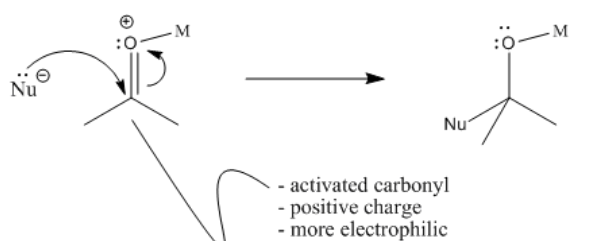Figure $$\PageIndex{2}$$: Coordination of an organic carbonyl to a metal ion makes the carbonyl more reactive towards nucleophiles.

A hydride attached to the metal can donate to the carbonyl. Remember, the hydride is nucleophilic, because the hydrogen atom is always more electronegative than a transition metal. Even the most electronegative transition metal, mercury, only has a Pauling electronegativity of 1.9, compared to 2.2 for hydrogen. When a hydride moves to the carbonyl from the metal, the carbonyl is turned into an alkoxide ligand.Figure $$\PageIndex{3}$$: The intermolecular reaction of a coordinated organic carbonyl with a coordinated nucleophile.

Notice that there is a difference between migratory insertion, or 1,1-insertion, and this beta-insertion, which is sometimes called a 1,2-insertion. Unlike migratory insertion, the nucleophile does not move to the atom attached to the metal. The nucleophile moves to the second atom away from the metal: the 2 position or the beta position.

Furthermore, beta-insertions of hydride ligands are easily reversible. An alkoxide ligand attached to a metal can easily lose a beta hydrogen and become a ketone or aldehyde.Figure $$\PageIndex{4}$$: The reverse of a 1,2-addition is a 1,2-elimination.
• In this case, the insertion can be reversible.
• The reverse of an insertion is called a 1,2-elimination or a beta-elimination.

The Greek lettering refers to the number of atoms away from the metal. The first atom attached to the metal is called the alpha position. A hydrogen on that atom is called an alpha hydrogen. The next atom along the chain is called the beta position. The third atom along the chain is the gamma position. A hydrogen attached to the beta position can undergo 1,2-elimination or beta-elimination.

• elimination leads to formation of a double bond.
• The double bond forms between the alpha and the beta position.

This nomenclature can be confusing because a carbonyl compound already has an alpha position and a beta position. The position is the carbonyl carbon and the position is the carbon next to the carbonyl. This Greek lettering system is a general way of designating positions and it is used in a number of different contexts; you need to be able to decide which context fits. If elimination is occurring, then the term, position, may mean one thing. If enolate formation is occurring, then the term, position, means something else.

Exercise $$\PageIndex{1}$$

Problem MI4.1.

In the following structures, identify any alpha, beta or gamma positions on the groups attached to the metal. In each case, show the potential products of 1,2-elimination of hydrogen.Figure $$\PageIndex{5}$$: Answer

Exercise $$\PageIndex{2}$$

Problem MI4.2.

Hydride transfer reduction is a method of converting aldehydes or ketones to alcohols. This catalytic reaction involves the use of a sacrificial alcohol as the solvent. As the aldehyde or ketone substrate is converted into alcohol, the alcohol solvent is converted into ketone. The mechanism is quite complicated, but at a simple level it could be imagined as taking place through a series of binding steps, eliminations and insertions.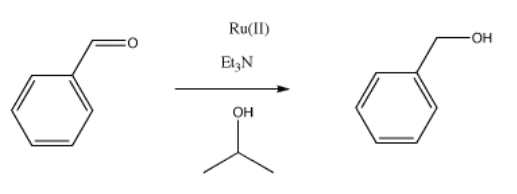Figure $$\PageIndex{6}$$:

Provide mechanisms based on the following descriptions for the reduction of benzaldehyde to benzyl alcohol.

1. The reaction involves binding of isopropanol or 2-propanol to a metal ion, such as Ru(II) or Ru2+. A deprotonation ensues, followed by elimination, and 2-propanone dissociates from the metal ion.
2. The ruthenium hydride formed in step (a) binds to the substrate, benzaldehyde. An insertion occurs, forming a metal alkoxide.
3. The metal alkoxide is protonated, and the resulting alcohol dissociates from the metal.
Answer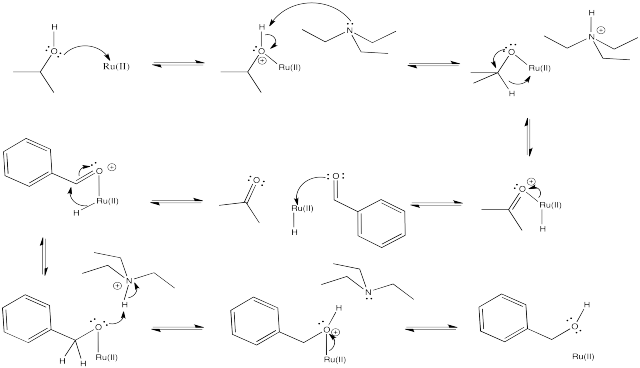Just like aldehydes and ketones, alkenes can undergo 1,2-insertions (also called beta hydride insertions). In terms of electrophiles and nucleophiles, this reaction is a little harder to imagine. However, we can still think of the hydride as a nucleophile. Maybe the alkene is an electrophile. Given that it is donating its pi electrons to the metal, we can think of it as "activated", a little bit like an activated carbonyl.

The formalisms of drawing a beta alkene insertion are tricky. If we use the metallacycle drawing of a bound alkene, it might look like this:Figure $$\PageIndex{7}$$:

More often, bound alkenes are drawn as shown as in the picture below. In that case, we could try to show the pi bond forming a new carbon-metal bond. The bond between the metal and alkene on the picture to the left does not really stand for a separate pair of electrons in this case; it just stands for the pi bond donating to the metal.Figure $$\PageIndex{8}$$:
• The reverse of elimination is insertion.
• A coordinated alkene is sometimes considered electrophilic because it is giving electrons to the metal.
• A coordinated alkene is activated, like a coordinated carbonyl compound.

So far, we have just looked at the 1,2-insertion of hydride ligands, but 1,2-insertions of alkyl ligands are also possible. In this case, an alkyl ligand would relocate onto the second atom in the former alkene. As a result, the alkyl chain attached to the metal would become two carbons longer.Figure $$\PageIndex{9}$$:

However, the opposite reaction, 1,2-elimination of an alkyl, is not very common. That reaction is usually restricted to movement of a hydrogen atom.Figure $$\PageIndex{10}$$:

Beta-insertion of hydride or alkyl ligands across alkenes is very important commercially. Many catalytic processes that are used to convert hydrocarbons into useful products depend on this reaction. Alkenes are readily made from petroleum or natural gas, so they are convenient starting materials to make more useful compounds. For example, ethene can be grown into longer carbon chains via a series of 1,2-alkyl insertions into coordinated ethene. This process is called "olefin oliomerization".Figure $$\PageIndex{11}$$:

That sequence of steps is crucial in the conversion of ethene into more valuable materials. It can be grown into hydrocarbon chains long enough to be used as fuels. However, more valuable products can be made such as soaps, detergents, and surfactants (via hydroformylation and other steps). In addition, the alkene may be functionalised in a variety of different ways if a long carbon chain needs to be incorporated into the synthesis of a more valuable commodity, such as a paint or a pharmacuetical.Figure $$\PageIndex{12}$$:

Exercise $$\PageIndex{3}$$

Provide the products of beta-elimination in each of the following cases. In each case, assume the elimination product is still bound to the metal.Figure $$\PageIndex{13}$$:Figure $$\PageIndex{14}$$: Answer

Exercise $$\PageIndex{4}$$

Provide products of the following beta-eliminations. In each case, assume the elimination product has already dissociated from the metal.Figure $$\PageIndex{15}$$:Figure $$\PageIndex{16}$$: Answer

Exercise $$\PageIndex{5}$$

Provide products of the following insertion reactions.Figure $$\PageIndex{17}$$: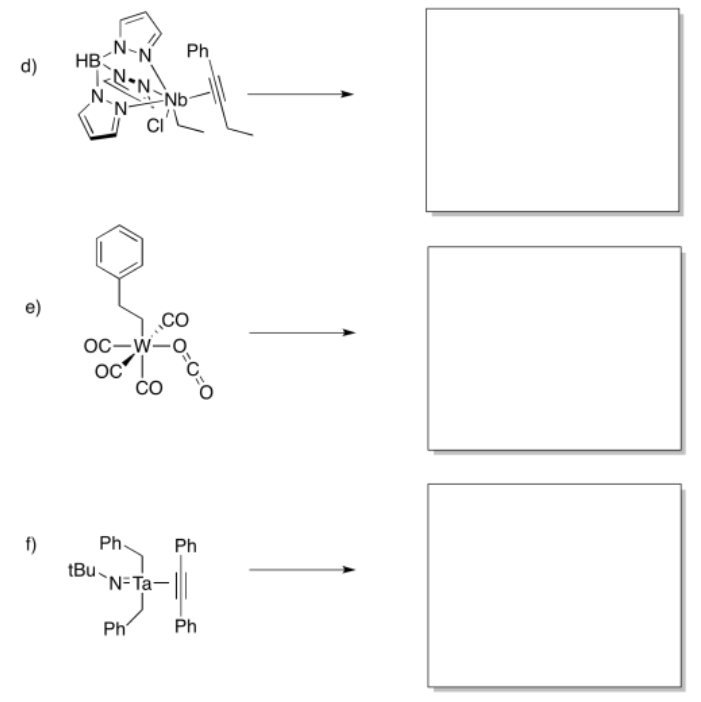Figure $$\PageIndex{18}$$:Figure $$\PageIndex{19}$$: Answer

Exercise $$\PageIndex{6}$$

Predict whether the following compounds will undergo 1,1-insertion, 1,2-insertion, or beta-elimination.Figure $$\PageIndex{20}$$:Figure $$\PageIndex{21}$$: Answer

Exercise $$\PageIndex{7}$$

Insertion and elimination reactions can also occur on solid metal surfaces. Often, solid metals are used as catalysts in industrial processes.

a) Copper adopts a face-centered cubic structure. Show a unit cell of copper on the following template.Figure $$\PageIndex{22}$$:

b) Alcohols such as ethanol can bind to the surface of the copper metal. Show an ethanol molecule binding to a copper in the unit cell.

c) The ethanol can undergo beta-elimination. Show the product of the reaction.

Answer a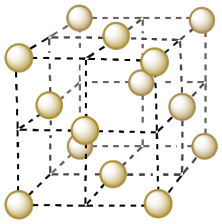Answer bAnswer c